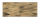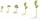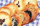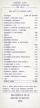# Fraction calculator

The calculator performs basic and advanced operations with fractions, expressions with fractions combined with integers, decimals, and mixed numbers. It also shows detailed step-by-step information about the fraction calculation procedure. Solve problems with two, three, or more fractions and numbers in one expression.

## Result:

### 2/5 * 31/2 + 4/5 = 11/5 = 2 1/5 = 2.2

Spelled result in words is eleven fifths (or two and one fifth).

### How do you solve fractions step by step?

1. Conversion a mixed number 3 1/2 to a improper fraction: 3 1/2 = 3 1/2 = 3 · 2 + 1/2 = 6 + 1/2 = 7/2

To find a new numerator:
a) Multiply the whole number 3 by the denominator 2. Whole number 3 equally 3 * 2/2 = 6/2
b) Add the answer from previous step 6 to the numerator 1. New numerator is 6 + 1 = 7
c) Write a previous answer (new numerator 7) over the denominator 2.

Three and one half is seven halfs
2. Multiple: 2/5 * 7/2 = 2 · 7/5 · 2 = 14/10 = 7 · 2/5 · 2 = 7/5
Multiply both numerators and denominators. Result fraction keep to lowest possible denominator GCD(14, 10) = 2. In the following intermediate step, cancel by a common factor of 2 gives 7/5.
In other words - two fifths multiplied by seven halfs = seven fifths.
3. Add: the result of step No. 2 + 4/5 = 7/5 + 4/5 = 7 + 4/5 = 11/5
For adding, subtracting, and comparing fractions, it is suitable to adjust both fractions to a common (equal, identical) denominator. The common denominator you can calculate as the least common multiple of both denominators - LCM(5, 5) = 5. In practice, it is enough to find the common denominator (not necessarily the lowest) by multiplying the denominators: 5 × 5 = 25. In the following intermediate step, the fraction result cannot be further simplified by canceling.
In other words - seven fifths plus four fifths = eleven fifths.

#### Rules for expressions with fractions:

Fractions - use the slash “/” between the numerator and denominator, i.e., for five-hundredths, enter 5/100. If you are using mixed numbers, be sure to leave a single space between the whole and fraction part.
The slash separates the numerator (number above a fraction line) and denominator (number below).

Mixed numerals (mixed fractions or mixed numbers) write as non-zero integer separated by one space and fraction i.e., 1 2/3 (having the same sign). An example of a negative mixed fraction: -5 1/2.
Because slash is both signs for fraction line and division, we recommended use colon (:) as the operator of division fractions i.e., 1/2 : 3.

Decimals (decimal numbers) enter with a decimal point . and they are automatically converted to fractions - i.e. 1.45.

The colon : and slash / is the symbol of division. Can be used to divide mixed numbers 1 2/3 : 4 3/8 or can be used for write complex fractions i.e. 1/2 : 1/3.
An asterisk * or × is the symbol for multiplication.
Plus + is addition, minus sign - is subtraction and ()[] is mathematical parentheses.
The exponentiation/power symbol is ^ - for example: (7/8-4/5)^2 = (7/8-4/5)2

#### Examples:

subtracting fractions: 2/3 - 1/2
multiplying fractions: 7/8 * 3/9
dividing Fractions: 1/2 : 3/4
exponentiation of fraction: 3/5^3
fractional exponents: 16 ^ 1/2
adding fractions and mixed numbers: 8/5 + 6 2/7
dividing integer and fraction: 5 ÷ 1/2
complex fractions: 5/8 : 2 2/3
decimal to fraction: 0.625
Fraction to Decimal: 1/4
Fraction to Percent: 1/8 %
comparing fractions: 1/4 2/3
multiplying a fraction by a whole number: 6 * 3/4
square root of a fraction: sqrt(1/16)
reducing or simplifying the fraction (simplification) - dividing the numerator and denominator of a fraction by the same non-zero number - equivalent fraction: 4/22
expression with brackets: 1/3 * (1/2 - 3 3/8)
compound fraction: 3/4 of 5/7
fractions multiple: 2/3 of 3/5
divide to find the quotient: 3/5 ÷ 2/3

The calculator follows well-known rules for order of operations. The most common mnemonics for remembering this order of operations are:
PEMDAS - Parentheses, Exponents, Multiplication, Division, Addition, Subtraction.
BEDMAS - Brackets, Exponents, Division, Multiplication, Addition, Subtraction
BODMAS - Brackets, Of or Order, Division, Multiplication, Addition, Subtraction.
GEMDAS - Grouping Symbols - brackets (){}, Exponents, Multiplication, Division, Addition, Subtraction.
Be careful, always do multiplication and division before addition and subtraction. Some operators (+ and -) and (* and /) has the same priority and then must evaluate from left to right.

## Fractions in word problems:

• Youth trackYouth track from Hronská Dúbrava to Banská Štiavnica which announced cancellation attracted considerable media attention and public opposition, has cost 6.3 euro per capita and revenue 13 cents per capita. Calculate the size of subsidies to a trip group o
• IssacIssac eats 1/6 of the pizza. Maya then eats 3/5 of the remaining pizza. What fraction of the original pizza is left?
• Golf ballsOf the 28 golf balls, 1/7 are yellow. How many golf balls are yellow? Use the model to help you. Enter your answer in the box.
• Bucket of clayTina and Bill share a 12-ounce bucket of clay. By the end of the week, Tina has used 1/6 of the bucket, and Bill has used 2/3 of the bucket of clay. How many ounces are left in the bucket?
• Magic bagEach time the prince crossed the bridge, the number of tolars in the magic bag doubled. But then the devil always conjured 300 tolars for him. When this happened for the third time, the prince had twice as much as he had in the beginning. How many tolars
• Father's gardenJohn's father has a garden in the back yard. The dimensions of the garden are shown here. A green rectangle is shown. The width is labeled as 2 and two-thirds feet. The length is labeled as 7 and three-fourths feet. Calculate the area of the garden. You f
• NumbersDetermine the number of all positive integers less than 4183444 if each is divisible by 29, 7, 17. What is its sum?
• SomeoneSomeone ate 1/10 of a cake, leaving only 9/10. If you eat 2/3 of the cake that is left, how much of a whole cake will you have eaten?
• SeedsOf the 72 wet seeds, 7/8 germinated. How many seeds germinate?
• A cookA cook wants to make enough cookies for 159 students. The cookie recipe makes 1 1/4 dozen cookies. A dozen equals 12. How many batches does the cook need to make if each student gets one cookie?
• Three friendsJohn, Peter, and Pablo each carried a 24 liters bucket full of water down the hill. After they reached the bottom, John's bucket was only 3/4 full, Peter's bucket was 2/3 full, and Pablo's was 1/6 full. How much liters of water did they spill altogether o
• Simplify expression with mixedWhen (7¹/3 + 2¾)÷(2 + 2¼ × 1¹/3) is simplified the result is?
• Price of stateEstimate the cost of the state based on following reason. State price is expressed as a percentage respectively share in the redistribution of national GDP. Employee creates monthly value € 868 (super-gross wage).Then tax him 35.2% employer contributions